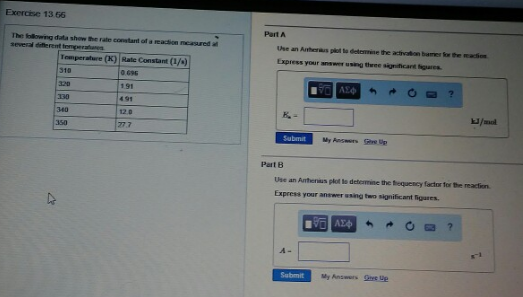# Problem: The following data show the rate constant of a reaction measured at several different temperatures. Part AUse an Arrhenius plot to determine the activation barrier for the reaction. Express your answer using three significant figures. Part BUse an Arrhenius plot to determine the frequency factor for the reaction. Express your answer using two significant figures.

###### FREE Expert Solution
100% (484 ratings)###### Problem Details

The following data show the rate constant of a reaction measured at several different temperatures.

Part A

Use an Arrhenius plot to determine the activation barrier for the reaction.

Part B

Use an Arrhenius plot to determine the frequency factor for the reaction.